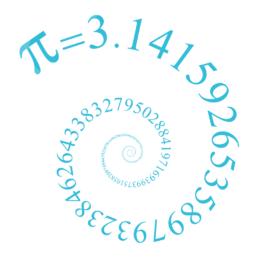Bournemouth Maths Tutor Barney M-T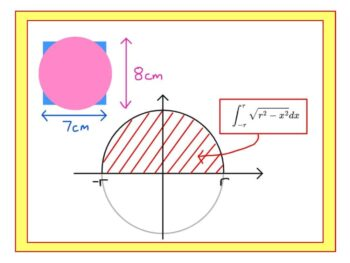WHAT’S THE POINT OF INTEGRATION BY SUBSTITUTION?

A PUZZLE: which of these shapes is larger: a square of side 7cm or a circle or diameter 8cm? Well that’s easy to answer if you know that the area of a circle is $A=\pi r^2$ but how do we know that $A=\pi r^2$ ?? Here is a particularly rigorous way that uses many of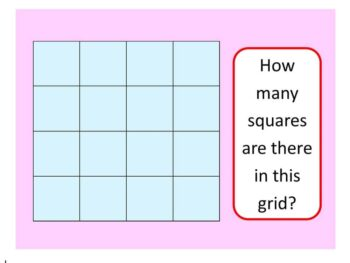HOW MANY SQUARES ARE THERE IN THIS GRID?

Before we see the quickest way to answer the question (scroll down if you are an expert!), let’s see some different possible approaches: GCSE APPROACH: There are 16 little squares.There is the one large square (the entire grid).But there are also some “2 by 2” squares, like the red one – I count 9 ofWHAT’S ZERO TIMES BY INFINITY?

SHORT ANSWER: 0 x ∞ = anything you like! Intrigued? Read on… LONG ANSWER: the question seems absurd: after all, zero multiplied by anything is zero, yet any multiple of infinity is always infinity. But 0 x ∞ cannot be both zero and infinity, can it? Infinity doesn’t behave in the same way as other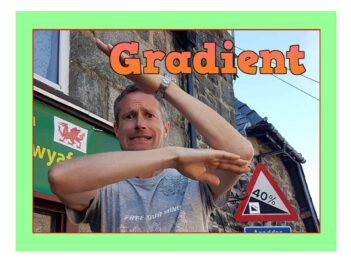Here I am at the World’s Steepest Road in Harlech, North Wales. The road sign indicates an almighty 40% – but how is this measured? ON ROADS: The 40% means that however far you travel horizontally, you travel 40% of that vertically. So if you go 100m across, you go 40m up. SO A ROAD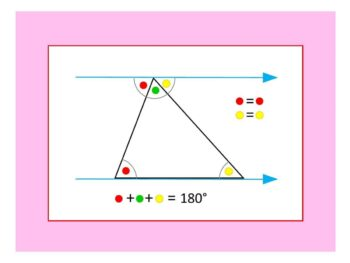WHY THE ANGLES IN A TRIANGLE ADD TO 180°

Given any triangle: Rotate so that the longest side lies horizontally along the base. Extend the base, and draw a parallel line going through the uppermost corner (these two lines are shown in light blue). The two red angles are the same size (using the well-known “parallel lines” angle result alternate angles, sometimes called “Z-angles“).PUZZLE OF THE WEEK

What shape goes in the intersection of this Venn Diagram? Read on for the answer: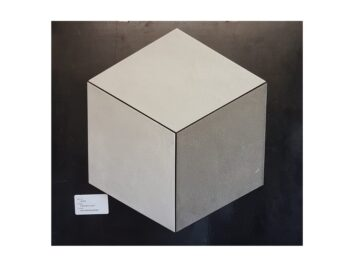GEOMETRY PUZZLE: WHAT SHAPE DO YOU SEE?

TRICKY TILINGS: Tiling stores are like treasure troves of geometrical amusement. Here’s a photo I took yesterday. The puzzle is simply: what shape(s) do you see? ANSWER: ….